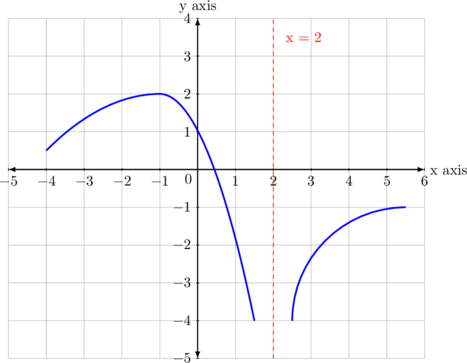# Problem Solving

## No 01

The given diagram shows the graph $$y = f(x)$$.The curve undergoes in succession, the following transformations:

• A translation of 3 units in the negative direction of the x-axis
• A scaling parallel to the x-axis by a factor $$\frac 12$$

Find the equation of the resulting curve in the form $$y = f(ax + b)$$ where a and b are constants to be found.

Sketch the graph of the resulting equation.